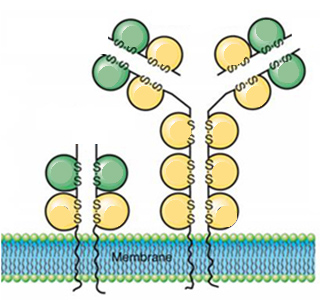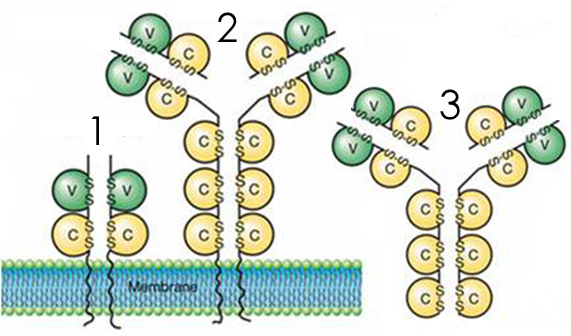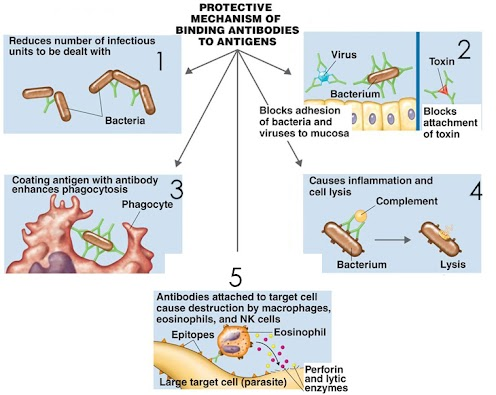Immune System Review
These questions MAY BE similar to those found on your lecture exam. THERE MAY BE MORE THAN ONE CORRECT ANSWER FOR ANY OF THE QUESTIONS BELOW.
1. Which of the following are considered a part of the innate (non-specific) defenses? *
1 point
Required
2. Which of the following are considered a part of the immune system (adaptable) defenses? *
1 point
Required
3. Which of the following are characteristics of the humoral branch of the immune system? *
1 point
Required
4. Which of the following are characteristics of the cell-mediated branch of the immune system? *
1 point
Required
5. Where are lymphocytes made? *
1 point
6. Which lymphocytes mature in the thymus? *
1 point
Required
7. Which lymphocytes mature in the bone marrow? *
1 point
Required
8. A lymphocyte that has never been activated by antigen is referred to as (a)_____. *
1 point
9. Which receptor binds Ag on B cells? *
1 point
10. Which receptor binds Ag on T cells? *
1 point
11. The green areas on the lymphocyte receptors below are _____. *
1 pointRequired
12. The yellow areas on the lymphocyte receptors below are _____.
1 point13. Which of the following is a T cell receptor? *
1 point14. Which of the following is a B cell receptor? *
1 point15. Which of the following is an Immunoglobulin? *
1 point16. Which of the following is an Antibody? *
1 point17. These molecules bind _____. *
1 point18. Where do naive B cells and T cells reside? *
1 point
19. How does antigen reach the naive B and T cells? *
1 point
20. Which of the following is the first step in lymphoid cell activation? *
1 point
21. Which of the following is the second step in lymphoid cell activation? *
1 point
22. Which of the following is the third step in lymphoid cell activation? *
1 point
23. Which of the following is the fourth step in lymphoid cell activation? *
1 point
24. Which of the following is the fifth step in lymphoid cell activation? *
1 point
25. Which of the following is the sixth step in lymphoid cell activation? *
1 point
26. When a B cell encounters Ag in the lymph node, this is an example of _____. *
1 point
27. When macrophages or dendritic cells bring Ag to a B cell, this is an example of _____. *
1 point
28. When a T cell activates a B cell, this is an example of _____. *
1 point
29. T cell independent B cell responses are *
1 point
Required
30. T cell dependent B cell responses are *
1 point
Required
31. How long does it take for a B cell to be activated? *
1 point
32. How long does it take for a B cell to respond to antigen? *
1 point
33. How do B cells respond to antigen? *
1 point
34. A primary response to infection can be detected in the blood by looking at _____. *
1 point
35. Immunological memory of prior infection can be detected in the blood by looking at _____. *
1 point
36. Number 1 below is an example of _____. *
1 point37. Number 2 below is an example of _____. *
1 point38. Number 3 below is an example of _____. *
1 point39. Number 4 below is an example of _____. *
1 point40. Number 5 below is an example of _____. *
1 point41. During antibody-dependent cell-mediated cytotoxicity, which of the following kills the target cell? *
1 point
42. Which of the following results of Ag-Ab interaction are effective against large parasites? *
1 point